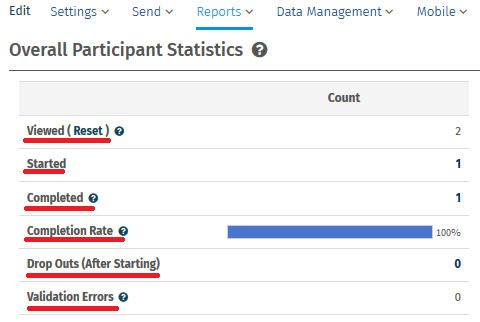# Statistical terms used in reports

## What do the various terms used in the real-time summary report or in the custom report mean?

• Viewed: Number of respondents who opened the survey link.
• Started: Number of respondents who have submitted a partial response however, not completed the survey.
• Completed: Number of respondents who completed the Survey
• Completion Rate: Percentage of respondents who completed the survey after starting.
• Drop Outs (After Starting): Number or respondents who did not finish the survey after starting.
• Average Time to Complete Survey: Average time taken by respondents to complete the survey.## Statistical terms.

• Mean:The mean is the arithmetic average of a set of values. Mean is calculated as the sum of all the given elements divided by the total number of elements.
• Confidence Interval: The confidence interval gives an estimated range of values which is likely to include an unknown population parameter, the estimated range being calculated for a given set of sample data collected. The width of the confidence interval gives us some idea about how uncertain we are about the unknown parameter. A very wide interval may indicate that more data should be collected before anything very definite can be said about the parameter.
• Standard Deviation: Standard deviation is a statistical measure of spread or variability. The standard deviation is the root mean square (RMS) deviation of the values from their arithmetic mean.
• Standard Error: The standard error is a method of measurement or estimation of the standard deviation of the sampling distribution associated with the estimation method.

War dieser Artikel hilfreich?
Das tut uns leid
Wie können wir es verbessern?